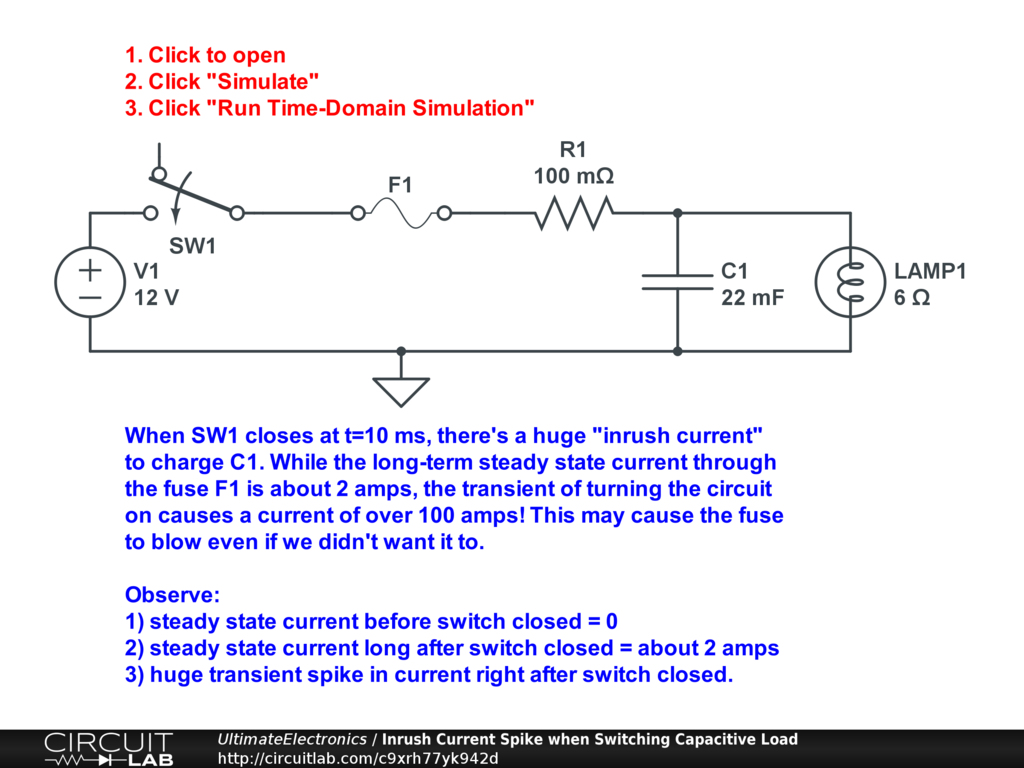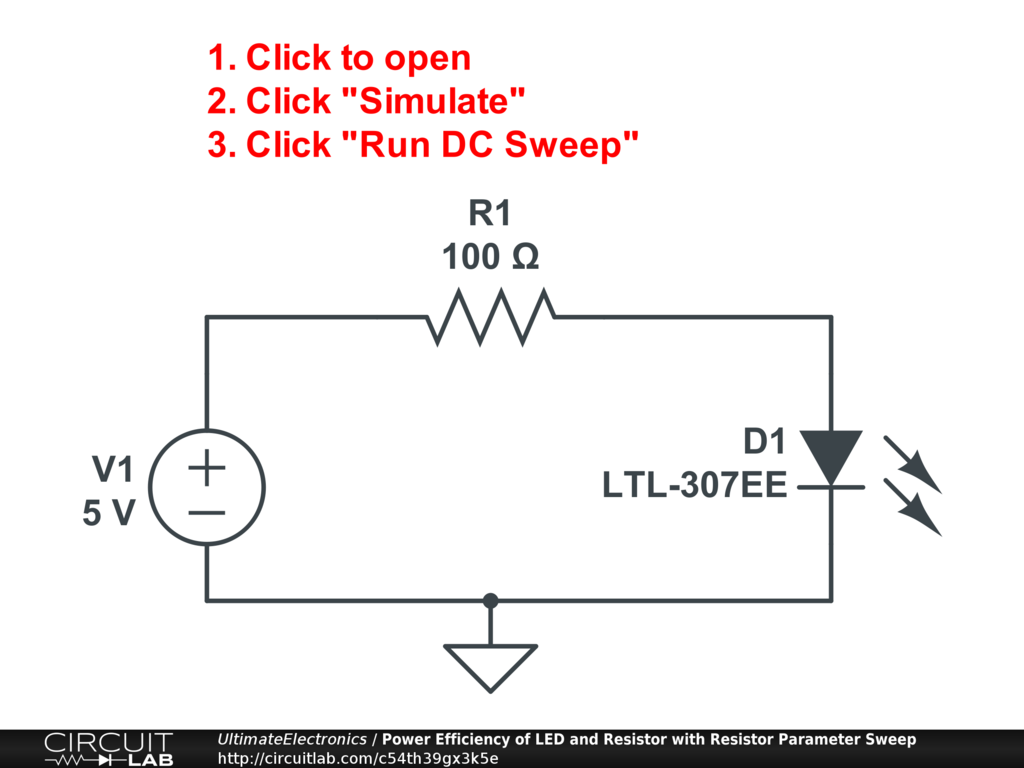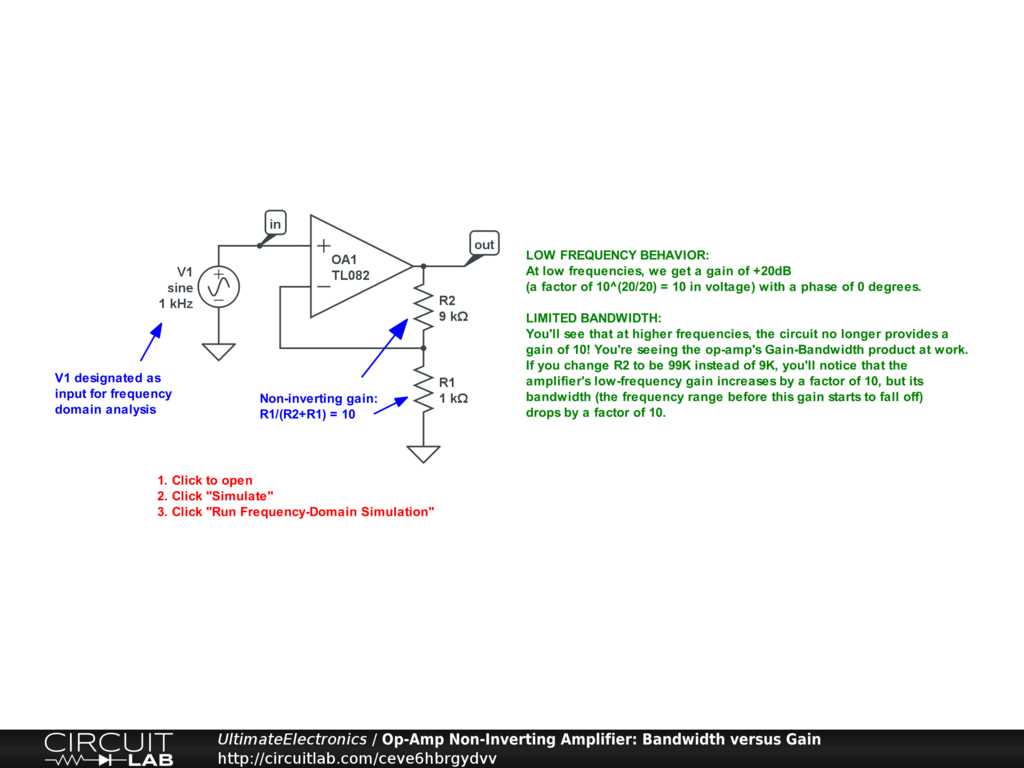← Prev: Introduction

Schematics & Simulations Tutorial

Whenever you see a schematic in this book, you can always click it to open the circuit in the CircuitLab software.

This will always open in a new browser tab so you can continue reading along.

Below are a few brief tutorials to walk you through the basic schematic drawing and circuit simulation features.

Basic Schematic ManipulationInteractive Exercise Click the circuit above and follow the steps below to quickly learn the user interface.

1. Click to open the circuit above.
2. To zoom the schematic:
• use the mousewheel, or
• use a two-finger drag up or down on your touchpad, or
• use the Ctrl-Plus or Ctrl-Minus keyboard shortcuts (Command-Plus or Command-Minus on macOS), or
• use the zoom in/out controls in the bottom right of the CircuitLab window, or
• use the Zoom In, Zoom Out, or Zoom to Fit options in the Edit menu
3. To pan around the schematic:
• hold the Ctrl (or Command) key while using your primary mouse button to drag, or
• hold the middle mouse button (press mousewheel) and drag, or
• switch to Pan mode using the bottom-left button within the CircuitLab window
4. To move a component:
• click and drag on voltage source V1, and move it a few grid lines to the left
• click and drag the bottom-most horizontal wire, and move it a few grid lines further down
• Notice that the software keeps the wires connected intelligently. To disable this feature if it's not doing what you'd like, just tap the Alt (or Option) button once while dragging.
5. To rotate a component:
• First, single-click on resistor R1 to select it.
• Then, either:
• press R to rotate clockwise, or
• press Shift+R to rotate counterclockwise, or
• right-click and use the Rotate CW or Rotate CCW options in the context menu
6. To flip a component:
• First, single-click on resistor R1 to select it.
• Then, either:
• press H to flip horizontally, or
• press V to flip vertically, or
• right-click and use the Flip Horizontally or Flip Vertically options in the context menu
7. To delete a component:
• First, single-click on resistor R1 to select it.
• Then, either:
• press Backspace or Delete to delete it, or
• use the Delete option in the Edit menu, or
• right-click and use the Delete option in the context menu
8. To undo:
• press Ctrl+Z (or Command+Z), or
• use the Undo option in the Edit menu
• right-click and use the Undo option in the context menu
9. To insert a component:
• First, use the mousewheel or two-finger scroll to scroll within the toolbox on the left until you find the component you'd like
• Then, either:
• drag the component from the toolbox to your schematic, or
• click the component in the toolbox, and then click in the schematic
10. To search for components:
• start typing in the search box at the top of the toolbox, or
• press / (frontslash) to bring keyboard focus to the search box
• enter "10k" to create a $10 \ \text{k}\Omega$ resistor, for example
• or, enter "2.2u" to create a $2.2 \ \mu\Omega$ capacitor, for example
11. To connect wires to your components:
• Hold your mouse over any terminal of any component. A gray circle will appear.
• Click and drag to draw a wire wherever you'd like it to go.
12. To modify a component's value or appearance:
• First, double-click on capacitor C1 to bring up the properties sheet, or
• right-click on capacitor C1 and use the Edit Parameters option in the context menu
• Change the DISP parameter, short for "DISPlay type", to "Polarized", and observe the change on the schematic.
• Change the C parameter, short for "Capacitance", to "22m / 2", and observe the change on the schematic.
• Finally, single-click on any blank space in the schematic to close the properties sheet.
13. To save your work, or generate schematic images as PDF, PNG, SVG, or EPS files:
• use the corresponding options under the File menu

DC Circuit SimulationInteractive Exercise Click the circuit above and follow the steps below to learn how to run DC circuit simulations.

1. Click to open the circuit above.
2. Click Simulate at the bottom of the screen.
3. Click the DC tab.
4. Click Run DC Solver.
5. Observe the list of outputs. These include:
• voltages
• currents
• power dissipation
• expressions like efficiency, a ratio of powers
6. Mouse over various wires and terminals in the schematic window. The voltage and current values will appear at the bottom-right.
7. Click any wires or terminals in the schematic to add those voltages and currents to the list of expressions in the simulation output.
8. Modify the circuit, and then re-run the DC simulation again.

DC Sweep SimulationInteractive Exercise Click the circuit above and follow the steps below to learn how to run DC sweep circuit simulations.

1. Click to open the circuit above.
2. Click Simulate at the bottom of the screen.
3. In the DC Sweep tab, look at the simulation settings:
• the Parameter is set to "V1.V", meaning the simulator should alter the voltage of voltage source V1 and use this as the independent variable on the x-axis of the plot.
• the Sweep Type is set to "Linear", meaning the simulator should try equally-spaced voltages.
• the Start is 0 and the End is 5, with a Step of 0.01, indicating that the simulator should these voltages: $0.00, 0.01, 0.02, \dots, 4.99, 5.00$
• the optional Second Parameter is enabled, set to try three different resistor values for the parameter "R1.R", the resistance of resistor R1: $95 \ \Omega, 100 \ \Omega, 105 \ \Omega$.
• the Outputs list has two expressions to plot:
• the first is "P(D1)/-P(V1)", which indicates the ratio of power consumed in diode D1 to the power supplied by V1.
• the second is "I(D1.nA)", indicating the current going into the anode of diode D1.
• You can add new outputs using the + Add Expression button.
• It's usually easier to add new outputs just by clicking various nodes and terminals on the schematic.
• the optional Advanced Graphing... window contains settings about how and what to plot.
4. Click Run DC Sweep. The simulation results are calculated and the results are plotted.
5. Mouse over any traces to see values along the curve.
6. Click and drag to zoom in to any region of the plot. Double click to zoom out again.
7. Pull out green horizontal and vertical markers from the edges of the graph windows to measure features of the plot.
8. Right click in the plot for a context menu.
9. At the top-right of the plot window, use Export Plot Images or Export Plot CSV to export simulation results.

Time-Domain SimulationInteractive Exercise Click the circuit above and follow the steps below to learn how to run time domain circuit simulations.

1. Click to open the circuit above.
2. Click Simulate at the bottom of the screen.
3. In the Time Domain tab, look at the simulation settings:
• the Start Time is almost always 0. Setting it to any higher number simply starts the plot later.
• the Stop Time is set to 50m, which means 50 milliseconds (0.050 seconds). Check out the Human-Friendly Inputs section of the documentation and the Orders of Magnitude, Logarithmic Scales, and Decibels section of this book on these metric prefixes.
• the Time Step is set to 10u, which means 10 microseconds (0.000010 seconds). This sets the resolution of the simulation.
• Choose a smaller time step number here for a higher-resolution simulation.
• Choose a larger time step for less processing time required to run the simulation.
• As a rule of thumb, a reasonable time step to start with is $\frac{1}{10} \ \text{to} \ \frac{1}{20}$ or so of the period of the highest frequency you're interested in. For example, if you have a circuit operating at 1000 Hz, which is a period of $\frac{1}{1000 \ \text{Hz}} = 1 \ \text{ms}$, you should aim for a time step of no more than 50u or 100u.
• Note that the Stop Time and Time Step also accept expressions. If you're looking at behavior at 60 Hz, for example, you can set the stop time to be "1/60 * 10" for ten for cycles, and the time step to be "1/60 / 20" for 1/20th of a cycle as a simulator time step.
• Another quick rule of thumb is to pick a time step about 1/1000th as long as your stop time.
• If you're not sure, start with more finely-grained timesteps for more accurate results.
• the Skip Initial is set to No. This is the normal behavior.
• Skip Initial = No is the default. It means that the simulator will first try to find a DC solution to cover the initial operating point state of the circuit at time t=0, before the time-domain simulation begins.
• Skip Initial = Yes should be used sparingly. It means that the simulator will treat all capacitors and inductors as discharged before $t=0$.
• The recommended way of controlling the initial conditions is to use switches, rather than the Skip Initial mode. Skip Initial tends to cause issues with more advanced components like transistors.
• the Outputs list has one expression to plot:
• "I(F1.nB)" indicates that the simulator should plot the current going into one of the terminals of the fuse F1.
• You can add new outputs using the + Add Expression button.
• It's usually easier to add new outputs just by clicking various nodes and terminals on the schematic.
• the optional Advanced Graphing... window contains settings about how and what to plot.
4. Click Run Time-Domain Simulation. The simulation results are calculated and the results are plotted.
5. Mouse over the blue trace to see values along the curve.
6. Click and drag to zoom in to any region of the plot. Double click to zoom out again.
7. Pull out green horizontal and vertical markers from the edges of the graph windows to measure features of the plot.
8. Right click in the plot for a context menu.
9. Right click on the "I(F1.nB)" within the plot legend to bring up options to calculate the integral, RMS, or average values of a signal. Adjust the green vertical markers to specify the time window over which to perform the calculation.
10. At the top-right of the plot window, use Export Plot Images or Export Plot CSV to export simulation results.

Frequency-Domain Simulation (Bode Plot)Interactive Exercise Click the circuit above and follow the steps below to learn how to run frequency domain circuit simulations.

1. Click to open the circuit above.
2. Click Simulate at the bottom of the screen.
3. In the Frequency Domain tab, look at the simulation settings:
• the Input is set to "V1", the name of a voltage source. For frequency-domain analysis, the input source must always be a voltage or current source in your circuit.
• the Start frequency is set to "1" Hz.
• the Stop frequency is set to "1e8" Hz, or 100 MHz. As above, you may also specify values like "100M" for 100 MHz, or "5G" for 5 GHz.
• the Points/Decade is set to "20". This will make calculations at twenty different points per decade of frequency, evenly spaced on a logarithmic frequency scale.
• Use more Points/Decade if you're studying a narrow-band phenomenon, like a resonance or high-Q filter. It can make sense to use 1000 points/decade or more in these situations.
• Use fewer Points/Decade to make the simulation calculations run faster if you need less resolution.
• As a rule of thumb, when in doubt, 20 points/decade is a good starting point.
• the optional Sweep Parameter is set to "R2.R", meaning the simulator should change the resistance of the resistor named R2.
• the Sweep Type is set to Custom, with two comma-separated values specified: "9k,99k", indicating the simulator should first simulate the circuit $R_2 = 9 \ \text{k}\Omega$, and should then try the circuit again with $R_2 = 99 \ \text{k}\Omega$. Both results will be plotted together for easy comparison.
• the Outputs list has two expressions to plot:
• "DB(MAG(V(out)))" indicates the magntiude of the output voltage signal, relative to the input, in a logarithmic decibel scale. +20dB would indicate the output voltage was 10X larger than the input, and +40dB would indicate 100X larger.
• "PHDEG(V(out))" indicates the phase angle, in degrees, of the output voltage signal, relative to the input. See the section on Complex Numbers for more.
• These two plots together are conventionally called a Bode plot.
• It's usually easier to add new outputs just by clicking various nodes and terminals on the schematic.
• the optional Advanced Graphing... window contains settings about how and what to plot.
4. Click Run Frequency-Domain Simulation. The simulation results are calculated and the results are plotted.
5. Mouse over the blue trace to see values along the curve.
6. Click and drag to zoom in to any region of the plot. Double click to zoom out again.
7. Pull out green horizontal and vertical markers from the edges of the graph windows to measure features of the plot.
8. Right click in the plot for a context menu.
9. At the top-right of the plot window, use Export Plot Images or Export Plot CSV to export simulation results.

What's Next

Return to the Table of Contents to continue with chapter 1, where we'll begin to explore the universal problem solving tools provided by the conceptual foundations of engineering.